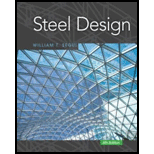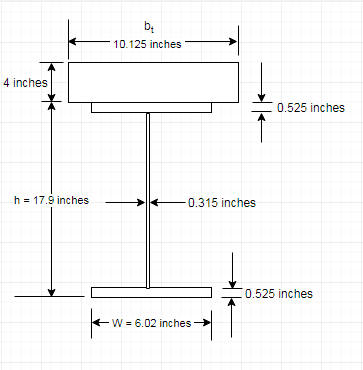Chapter 9, Problem 9.1.1P### Steel Design (Activate Learning wi...

6th Edition
Segui + 1 other
ISBN: 9781337094740

#### Solutions

Chapter
Section### Steel Design (Activate Learning wi...

6th Edition
Segui + 1 other
ISBN: 9781337094740
Textbook Problem

# A W 18 × 40 floor beam supports a 4-inch-thick reinforced concrete slab with an effective width b of 81 inches. Sufficient anchors are provided to make the beam fully composite. The 28-day compressive strength of the concrete is f c ' = 4 ksi.a. Compute the moment of inertia of the transformed section.b. For a positive service load moment of 290 ft-kips, compute the stress at the top of the steel (indicate whether tension or compression), the stress at the bottom of the steel, and the stress at the top of the concrete.

To determine

(a)

The moment of inertia of the transformed section.

1761.00(inches)4

Explanation

Given:

A W 18 X 40 floor beam, the 28-day compressive strength of the concrete is fC'

4ksi, 4-inch-thick reinforced concrete slab with an effective width b of 81 inches.

Calculation:

We have the modulus of elasticity of concrete as follows:

EC=(wC)32fC'ksi

Where, the modulus of elasticity of concrete is EC,

unit weight of concrete is wC and

the 28-day compressive strength of concrete is fC'.

Substitute 145lbft3 for wC and 4ksi for fC'.

EC=(145lbft3)324ksiEC=1746.03lbft3×2ksiEC=3492.06ksi.

Modular ratio by using the following formula:

n=ESEC

Where, the modulus of elasticity of concrete is EC,

the modulus of elasticity of steel is ES,

and n is the modular ratio.

n=ESEC

Substitute 3492.06ksi for EC and 29000ksi for ES.

n=29000ksi3492.06ksin=8.3048

Now the section will be transformed.

Since the modulus of elasticity of concrete can only be approximated, the usual practice of rounding n to the nearest whole number is sufficiently accurate. Thus,

The transformed width of the section is as following:

bt=bn

Where, bt is the transformed width,

b is the width of the section and

n is the modular ratio.

Substitute 81 for b and 8 for n.

bt=818bt=10.125in

Following is the section for the given set of conditions:Data from the steel code:

 DesignationImperial (in x lb/ft) Depthh (in) Widthw (in) Web Thicknesstw (in) Flange Thicknesstf (in) Sectional Area (in2) Weight (lbf/ft) Static Parameters Moment of Inertia Elastic Section Modulus Ix (in4) Iy (in4) Sx (in3) Sy (in3) W 18 x 40 17.9 6.02 0.315 0.525 11.8 40 612 19.1 68.4 6.4

The transformed section is shown in the above figure. The neutral axis is not known yet whether it lies in the steel or the concrete.

The location of the neutral axis can be found by applying the principle of moments with the axis of moments at the top of the slab. The computations are summarized in Table, and the distance from the top of the slab to the centroid is:

 Component A(inches)2 Y(inches) AY(inches)3 Concrete 40.5 2.0 81.0 W 18 X 40 11.8 12.95 152.81 Sum 52.3 233.81

The distance of centroid of the section is as follows:

Y¯=A×yA

Substitute 233.81(inches)3 for A×y and 52.3(inches)2 for A

Y¯=233.81(inches)352.3(inches)2.Y¯=4.4705inches.

Since this is greater than 4 inches (the thickness of the slab) the neutral axis lies below the slab in the web. Applying the parallel axis theorem and tabulating the computations in table, we obtain the moment of inertia of the transformed section as:

 Component A(inches)2 Y(inches) I¯(inches)4 d =Y¯−Y(inches) Itransformed=I¯+Ad2(inches)4 Concrete 40.5 2.0 54 2.4705 301 W 18 X 40 11.8 12.95 612 8.4795 1460 Sum 52.3 1761

Conclusion:

Therefore, total moment of inertia of the transformed section is 1761.00

(inches)4.

To determine

(b)

The stress at the top of the steel (indicate whether tension or compression), the stress at the bottom of the steel, and the stress at the top of the concrete.

fs=0.927ksi(compressive)_,fs=34.44ksi(Tensile)_andfs=1.104ksi(compressive)_.

Explanation

Given:

Positive service load moment of 290 ft-kips.

Calculation:

For the computation of the stress at top of the steel is as following:

fs=MyItransformed

Where, the stress at the top of the steel is fS, the moment acting on the composite section is M, the distance from neutral axis to the top of steel is Y and the moment of inertia of the transformed section is I.

The distance from neutral axis to top of steel section is as follows:

y=y¯ty=4.47054y=0.4705

Here the thickness of the concrete slab is t.

Compute the stress at top of the steel as:

fs=M(y¯t)Itransformedfs=(290×12)in.kips(0.4705)in1761.00in4fs=0.927ksi.

As, the centroid lies below the top of the steel, the stress is compressive.

Now, the stress at the bottom of the steel is as follows:

fs=MyItransformed

The distance from the neutral axis to bottom of steel section.

y=dw+ty¯y=17.9+44.4705y=17.43.

Where, dw is the depth of the web.

fs=M(dw+ty¯)Itransformedfs=(290×12)in.kips(17.43)in1761.00in4fs=34.44ksi.

As, the centroid lies above the top of steel, the stress is tensile.

Stress at the top of the slab is as follows:

fs=MynItransformed

y=y¯

Where, n is the modular ratio is n.

fs=(290×12)in.kips(4.4705)in8×1761.00in4fs=(15557.34)in2.kips14088.00in4fs=1.104ksi.

As the concrete slab is above the neutral axis, hence the stress is compressive.

Conclusion:

Therefore, the stress at top of steel section is fs=0.927ksi (compressive), the stress at bottom of steel section is fs=34.44ksi (Tensile) and the stress at the top of the slab is fs=1.104ksi (compressive).

Find quality solutions to thousands of textbooks. All for just \$9.99/month

Get As ASAP

## Additional Engineering Solutions

#### Explain the principles of adaptive cruise control.

Automotive Technology: A Systems Approach (MindTap Course List)

#### What machine tool produces cylindrical parts?

Precision Machining Technology (MindTap Course List)

Find all the answers to your study problems with bartleby.
Textbook solutions plus Q&A. Get As ASAP arrow_forward